## Monday, January 28, 2008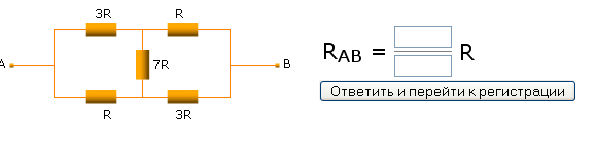A few weeks ago , I brought up a Russian CAPTCHA that asks to find resistance between end-points in a version of Wheatstone bridge in order to access a forum

There's been some discussion of this problem on Reasonable Deviations blog, with Vladimir Zacharov writing up a general solution for this circuit using two Kirchhoff's (Kirkhoff) laws and one Ohm's law directly .

For larger circuits, this approach gets exponentially more tedious since you get an equation for every loop in the circuit. But apparently you can ignore most of them and look only at "fundamental loops".

An alternative approach is to use one Kirchhoff law and one Ohm's law. Note that:
1) Current across an edge is proportional to voltage drop divided by resistance
2) Net current at each node is 0, (not counting external current)

Hence, for net current at i'th node we get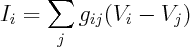Here g's are conductances on edges. This is just a set of linear equations, so you can rewrite them as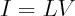where L is the combinatorial Laplacian of the graph with conductances being edge weights. We inject 1 Amp at node 1 (A), extract 1 Amp at node 4 (B), and solve for voltages.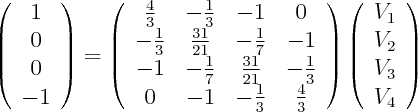Use your favourite method to solve this linear system. For instance, the following is a solution: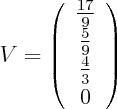Since current is 1, voltage drop is the same as resistance, hence 17/9 is the answer.

Linear resistor networks turn out to be an amazingly rich subject with connections to many other areas, as can be seen from other ways of solving this problem

• Combinatorics 1: add an edge from 1 to 4 with conductance 1. Resistance is the weighted sum of spanning trees containing new edge divided by the weighted sum of all spanning trees (weighted by product of edge conductances). Formula 4.27 of Wu

• Combinatorics 2: resistance is the determinant of combinatorial Laplacian with 1st/4th row/column removed divided by determinant of said Laplacian with 1st column/row removed. Formula 6 of Bapat and 4.34 of Wu

• Probability Theory 1: Resistance is 1/(cd) where c is conductance of node 1 (sum of incoming edge conductances), d is the probability of random walker starting at node 1 reaching node 4 before coming back to node 1, with node transition probabilities defined by formula 12 in Klein. Formula 13 of Klein

• Probability Theory 2: Add an edge from 1 to 4 with conductance 1. Resistance is the odds of random spanning tree on the graph containing this edge, where probability of each spanning tree is proportional to the product of edge conductances. Follows from formula 4.27 of Wu

• Markov Chain simulation: create a Markov chain with 1 and 4 being trapping states, and transition probabilities defined in previous method. Start with all probability in state 1, let the mass escape once, then simulate Markov chain till convergence. Resistance is 1 divided by mass in node 4 after convergence. Section 1.2.6 of Doyle

• Circuit transformation rules: use star-triangle, parallel and series laws to simplify the circuit to a single resistor. Page 44 of Bollobas. Note that two of these transformation rules have an analogue in graphical models, sections 4.4,4.5 of Sokal.

• Optimization Theory: Resistance is R in the following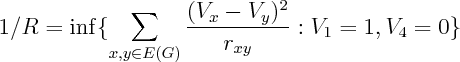Corollary 5, Chapter 9 of BollobasAnonymous said...

Very cool, Yaroslav. I always love seeing all the ways of solving a problem.

Abir said...

These captcha is really changing its way of using as there has so many new way to use captcha in recent time which people love to. site that helps me a lot on my academic papers writing.

sophie said...

Russian Captcha revisited is I think about electric circuits but I have seriously no interest in it.All the electrical engineers I want you guys to follow the link.Thanks for sharing this post.

Milo Anderson said...

I am really happy to see this post as it looked me very informative and I left my http://business.proposalwritingservice.com// work pending for this.Keep shaing more posts like this.Thank you so much.

Milo Anderson said...

This time your topic is quite different and I would recommend my sister to click site for all related details.I like such topics.Keep posting more like this.

Kenneth M. Lundgren said...

Captcha is a process to confirm that your are a human in any website registration or important login. This is very good article about Russian Captcha introduced with valuable information. Also check our essay paraphrasing tools from https://www.paraphrasingonline.com/rephrase-my-essay. This information should helpful for Internet workers. Thanks

draj said...

Excellent machine learning blog,thanks for sharing...
Seo Internship in Bangalore
Smo Internship in Bangalore
Digital Marketing Internship Program in Bangalore

john said...